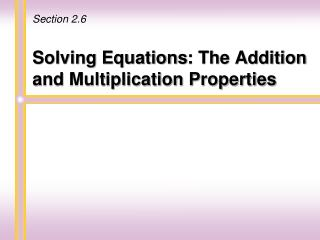# Solving Equations : The Addition and Multiplication Properties - PowerPoint PPT PresentationDownload PresentationSolving Equations : The Addition and Multiplication Properties

Solving Equations : The Addition and Multiplication PropertiesDownload Presentation## Solving Equations : The Addition and Multiplication Properties

- - - - - - - - - - - - - - - - - - - - - - - - - - - E N D - - - - - - - - - - - - - - - - - - - - - - - - - - -
##### Presentation Transcript

1. Section 2.6 Solving Equations: The Addition and Multiplication Properties

2. Equation Statements like 5 + 2 = 7 are called equations. An equation is of the form expression = expression An equation can be labeled as Equal sign x+ 5 = 9 left side right side Martin-Gay, Prealgebra, 5ed

3. Solving/Solution When an equation contains a variable, deciding which values of the variable make an equation a true statement is called solving an equation for the variable. A solution of an equation is a value for the variable that makes an equation a true statement. Martin-Gay, Prealgebra, 5ed

4. Solving/Solution ... Determine whether a number is a solution: Is -2 a solution of the equation 2y + 1 = -3? Replace y with -2 in the equation. 2y + 1 = -3 ? 2(-2) + 1 = -3 ? - 4 + 1 = -3 -3 = -3 True Since -3 = -3 is a true statement, -2 is a solution of the equation. Martin-Gay, Prealgebra, 5ed

5. Solving/Solution ... Determine whether a number is a solution: Is 6 a solution of the equation 5x - 1 = 30? Replace x with 6 in the equation. 5x - 1 = 30 ? 5(6) - 1 = 30 ? 30 - 1 = 30 29 = 30 False Since 29 = 30 is a false statement, 6 is not a solution of the equation. Martin-Gay, Prealgebra, 5ed

6. Solving/Solution... To solve an equation, we will use properties of equality to write simpler equations, all equivalent to the original equation, until the final equation has the form x= number or number =x Equivalent equations have the samesolution. The word “number” above represents the solution of the original equation. Martin-Gay, Prealgebra, 5ed

7. Addition Property of Equality Let a, b, and c represent numbers. If a =b, then a+c=b+c and a – c = b - c In other words, the same number may be added to or subtracted from both sides of an equation without changing the solution of the equation. Martin-Gay, Prealgebra, 5ed

8. Solve for x. x- 4 = 3 To solve the equation for x, we need to rewrite the equation in the form x= number. To do so, we add 4 to both sides of the equation. x- 4 = 3 x- 4 + 4= 3 + 4 Add 4 to both sides. x= 7 Simplify. Martin-Gay, Prealgebra, 5ed

9. Check x- 4 = 3 Original equation 7- 4 = 3 Replace x with 7. 3 = 3 True. Since 3 = 3 is a true statement, 7 is the solution of the equation. To check, replace x with 7 in the original equation. ? Martin-Gay, Prealgebra, 5ed

10. Helpful Hint Remember to check the solution in the original equation to see that it makes the equation a true statement. Martin-Gay, Prealgebra, 5ed

11. Helpful Hint Remember that we can get the variable alone on either side of the equation. For example, the equations x= 3 and 3 =x both have a solution of 3. Martin-Gay, Prealgebra, 5ed

12. Multiplication Property of Equality Let a, b, and c represent numbers and let c 0. If a=b, then ac=bc and In other words, both sides of an equation may be multiplied or divided by the same nonzero number without changing the solution of the equation. Martin-Gay, Prealgebra, 5ed

13. Solve for x 4x= 8 To solve the equation for x, notice that 4 is multiplied by x. To get x alone, we divide both sides of the equation by 4 and then simplify. 1x = 2 or x = 2 Martin-Gay, Prealgebra, 5ed

14. Check To check, replace x with 2 in theoriginal equation. 4x= 8 Original equation 4 2= 8 Let x=2. 8 = 8 True. The solution is 2. ? Martin-Gay, Prealgebra, 5ed

15. Helpful Hint As reviewed in Chapter 1, don’t forget that order is important when subtracting. Notice the translation order of numbers and variables below. Phrase a number less 9 a number subtracted from 9 Translation x - 9 9 - x Martin-Gay, Prealgebra, 5ed